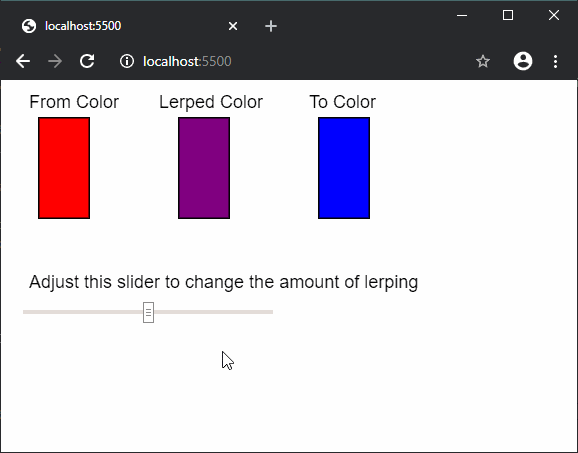GeeksforGeeks App
Open AppBrowser
Continue

## Related Articles

The lerpColor() function is used to interpolate two colors to find a third color between them. The amount of interpolation between the two colors can be set using the amt parameters. The color interpolation depends on the current color mode.

Syntax:

`lerpColor(c1, c2, amt)`

Parameters: This function accepts three parameters as mentioned above and described below:

• c1: It is a p5.Color which represents the first color from which the final color will be interpolated.
• c2: It is a p5.Color which represents the second color to which the final color will be interpolated.
• amt: It is a number between 0 and 1 which determines which color will be used more for the interpolation. A value near 0.1 would prefer the first color more and a value near 0.9 would prefer the second color for interpolation.

Return Value: It returns a p5.Color element with the interpolated color.

The example below illustrate the lerpColor() function in p5.js:

Example:

 `function` `setup() {``  ``createCanvas(500, 350);``  ``textSize(18);``   ` `  ``text(``"From Color"``, 20, 20);``  ``fromColor = color(``"red"``);`` ` `  ``text(``"Lerped Color"``, 150, 20);`` ` `  ``text(``"To Color"``, 300, 20);``  ``toColor = color(``"blue"``);``   ` `  ``text(``"Adjust this slider to change the"``+``             ``" amount of lerping"``, 20,  200)``  ``alphaSlider = createSlider(0, 100, 50);``  ``alphaSlider.position(20, 220);``  ``alphaSlider.style(``'width'``, ``'250px'``);``}`` ` `function` `draw() {``  ``lerpedColor = lerpColor(fromColor, toColor, alphaSlider.value() / 100);`` ` `  ``fill(fromColor);``  ``rect(30, 30, 50, 100);`` ` `  ``fill(lerpedColor);``  ``rect(170, 30, 50, 100);`` ` `  ``fill(toColor);``  ``rect(310, 30, 50, 100);``}`

Output:Online editor: https://editor.p5js.org/

My Personal Notes arrow_drop_up
Related Tutorials